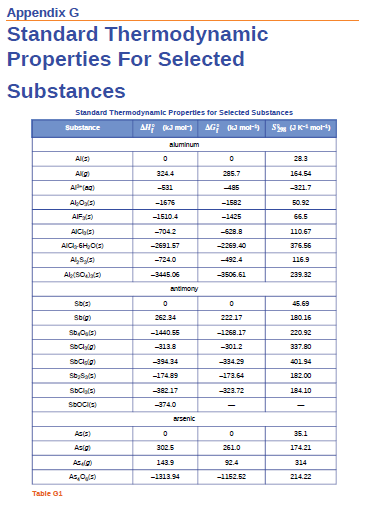Chemistry Practice Problems Entropy Practice Problems Solution: Use the standard entropy data in Appendix G to det...

⚠️Our tutors found the solution shown to be helpful for the problem you're searching for. We don't have the exact solution yet.

# Solution: Use the standard entropy data in Appendix G to determine the change in entropy for each of the reactions listed below. All the processes occur at the standard conditions and 25 °C.(a) MnO2(s) ⟶ Mn(s) + O2(g)(b) H2(g) + Br2(l) ⟶ 2HBr(g)(c) Cu(s) + S(g) ⟶ CuS(s)(d) 2LiOH(s) + CO2(g) ⟶ Li2CO3(s) + H2O(g)(e) CH4(g) + O2(g) ⟶ C(s, graphite) + 2H 2O(g)(f) CS2(g) + 3Cl2(g) ⟶ CCl4(g) + S2Cl2(g)

###### Problem

Use the standard entropy data in Appendix G to determine the change in entropy for each of the reactions listed below. All the processes occur at the standard conditions and 25 °C.

(a) MnO2(s) ⟶ Mn(s) + O2(g)
(b) H2(g) + Br2(l) ⟶ 2HBr(g)
(c) Cu(s) + S(g) ⟶ CuS(s)
(d) 2LiOH(s) + CO2(g) ⟶ Li2CO3(s) + H2O(g)
(e) CH4(g) + O2(g) ⟶ C(s, graphite) + 2H 2O(g)
(f) CS2(g) + 3Cl2(g) ⟶ CCl4(g) + S2Cl2(g)Entropy

Entropy

#### Q. By calculating ΔSuniv at each temperature, determine if the melting of 1 mole of NaCl(s) is spontaneous at 500 °C and at 700 °C.What assumptions are m...

Solved • Thu Nov 29 2018 10:10:15 GMT-0500 (EST)

Entropy

#### Q. Using the relevant S°298 values listed in Appendix G, calculate ΔS°298 for the following change:(a) N2(g) + 3H2(g) ⟶ 2NH3(g)

Solved • Thu Nov 29 2018 09:55:04 GMT-0500 (EST)

Entropy

#### Q. “Thermite” reactions have been used for welding metal parts such as railway rails and in metal refining. One such thermite reaction is Fe2 O3(s) + 2Al...

Solved • Thu Nov 29 2018 09:45:18 GMT-0500 (EST)

Entropy

#### Q. Determine the entropy change for the combustion of gaseous propane, C 3H8, under the standard conditions to give gaseous carbon dioxide and water.

Solved • Thu Nov 29 2018 09:42:42 GMT-0500 (EST)